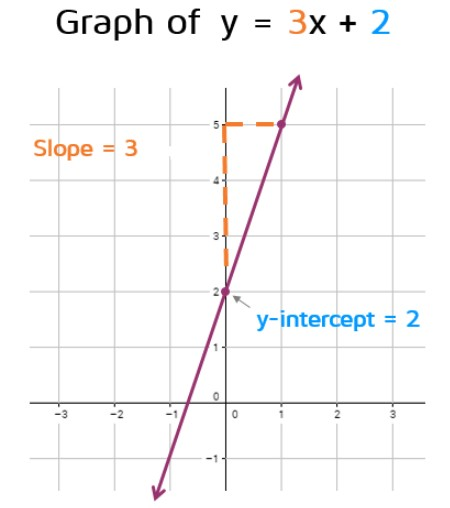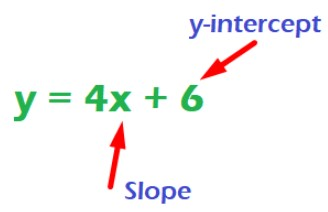# Slope intercept form Calculator

Enter the values in the below calculator. Hit the Calculate button to get the slope intercept form of a line using y=mx+b equation.

Give Us Feedback

Slope intercept form calculator is an online tool that is used to find slope intercept form (equation of line) using two points, y-intercept, or one point and slope.

## What is slope intercept form?

Slope intercept is a form of linear equation that can be used to find the equation of a straight line with y intercept and slope of line.## Slope intercept formula

The slope intercept equation can be represented as:

y = mx + b

Where,

• x, y represents the x and y coordinates,
• m is slope of line, and
• b is y intercept.

The equation of slope intercept varies in USA and UK. In UK, variable c is used to represent y-intercept.

y = mx + c

## How to find the slope intercept form (equation of a straight line)?

To find equation of a straight line with slope and y-intercept, follow the example below.

Example:

Find the Straight line equation if y-intercept is 6 and slope is 4.

Solution:

Step 1: Identify the values.

b = 6

m = 4

Step 2: Write the slope form equation and place the values.

y = mx + b

y = 4x + 6If you need to calculate the slope using two points, use slope calculator.

## Examples of Slope Intercept form

 Questions Results Points of line: (3, -4) (12, 6) Answery = 1.11x - 7.33Step-by-step solution:Step 1: Solve for slope "m".m = (y2 - y1)/(x2 - x1)m = (6 - (-4))/(12 - 3) = 10/9m = 1.11Step 2: Solve for y-intercept "b".y = mx + b6 = 1.11(12) + bb = -7.33Step 3: Slope intercept formy = 1.11x + (-7.33)y =1.11x - 7.33 slope = 15 and point = (6, -12) Answery = 15x - 102Step-by-step solution:Step 1: Solve to find "b".y = mx + b-12 = 15(6) + bb = -102Step 2: Slope intercept form.y = mx + by = 15x + (-102)y = 15x - 102 Slope = m = 9y-intercept form = 6 Answery = 9x + 6Step-by-step solution:substitute slope and y-intercept form to the general equation of slope intercept form to calculate it.y = mx + by = 9x + 6 Coordinate point:(-3, 12) and (6.-8) Answery = -2.22x +5.34Step-by-step solution:Step 1: Solve for slope "m".m = (y2 - y1)/(x2 - x1)m = (-8 - 12))/(6 - (-3)) = -20/9m = -2.22Step 2: Solve for y-intercept "b".y = mx + b-8 = -2.22(6) + bb = 5.34Step 3: Slope intercept formy = -2.22x + 5.34

### References

1. Khan Academy. (n.d.). Intro to slope-intercept form (Y=mx+b) | algebra (video). Retrieved january 4, 2020 from Khan Academy.
2. Alamo.edu. (2020). Slope Intercept Formula. Retrieved 4 january 2020.

### Math Tools